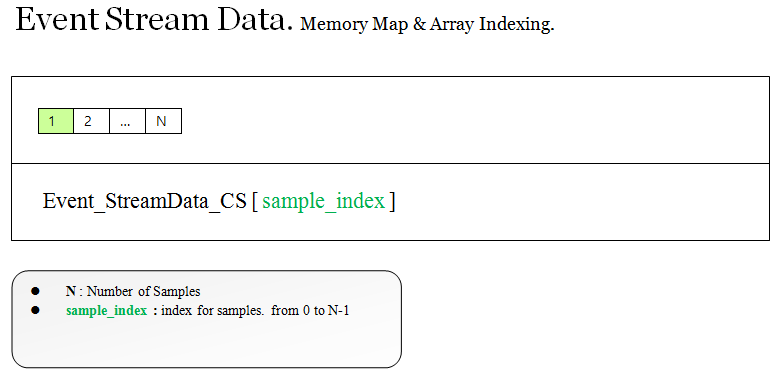### struct : ST_STREAMDATA_LXDAPI

LXDeviceAPI.h provides the typedef of ST_STREAMDATA_LXDAPI.

```// struct for stream. LXDeviceAPI.h

typedef struct _stStreamData_LXDAPI
{
...
unsigned int*	Event_StreamData_CS; // array for event marking

double*	Wave_StreamData_CS;  // array for measured multi channel bio signal
...

}ST_STREAMDATA_LXDAPI;```

The most important member variables are  Wave_StreamData_CS for multi channel bio signal and Event_StreamData_CS for event marking.

### Usages

Declare the variable for stream data type and feed into OpenDevice as a address of variable.

```ST_STREAMDATA_LXDAPI stStreamData;
int NumSampleReturn = 32 ; //available range 1~ 128. the number of samples to get the stream data per each message from LXDeviceAPI.
OpenDevice_LXDeviceAPI( , &stStreamData,NumSampleReturn , );```

### Dynamic Memory Allocation by LXDeviceAPI

LXDeviceAPI dynamic allocates the memory for arrays when you call the OpenDevice. The size of memory is determined by the OpenDevice’s parameter `int numsample_return ` which is the number of samples per each message  received.  LXDeviceAPI deletes the memory if CloseDevice is called.

### Memory Map, Array Indexing.

The array Wave_StreamData_CS has real time measured sampling data from the device.

The number of samples is determined by a parameter  `int numsample_return` when you call the OpenDevice.The Event_StreamData_CS has a value of “event id” which is the same as a parameter `unsigned int event_id` when you call the EventMarkingOnStream. The number of samples is the same as that of Wave_StreamData_CS.### Retrieving the one sample from Stream Array

#### Array indexing for wave data

The following code shows how to get the one sample for specific channel index and sample index,

```double one_sample_wave = stStreamData.Wave_StreamData_CS[sample_index + NumSampleReturn * channel_index ]

```

where,

• channel_index  : Available value range form 0 to ( number of channel   – 1). The number of channel is specific to device. In the case of  QEEG-64FX, the number of channel is 67 ( EEG 64ch + Bipolar 3ch).
• sample_index : Available value range from  0 to (NumSampleReturn – 1). The NumSampleReturn is determined by the parameter `int numsample_return` when you call the OpenDevice.

#### Array indexing for event data.

```unsigned int one_sample_event = stStreamData.Event_StreamData_CS[ sample_index ]

```

where,

• sample_index : Available value range from  0 to (NumSampleReturn- 1).The NumSampleReturn is determined by the parameter `int numsample_return` when you call the OpenDevice.

#### Indexing Example

Array indexing example for two channel signals and event. The chart plots the both signals and event simultaneously.